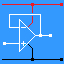RANDOM PAGE

SITE SEARCH

LOG
IN

HELP

# Op Amps - Key Facts

This is the AQA version closing after June 2019. Visit the the version for Eduqas instead.

 Everyone Ideal Op Amp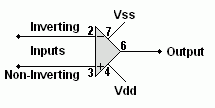Gain Infinity Input resistance Infinity Output resistance Zero Gain Bandwidth Product (GBP) Infinity Gain x Frequency = GBP Everyone Comparator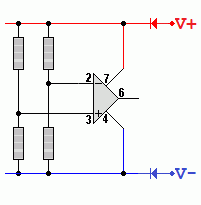Gain Infinity Output Always saturated high or low Input Resistance Infinity Comparisons If V+ is greater than V- then the output is high. If V+ is less than V- then the output is low. AS A2 Inverting Amplifier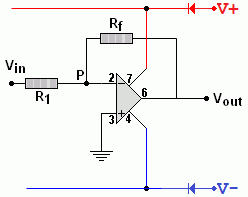Gain Gv = -Rf / R1 Gv = Vout / Vin Input resistance R1 Virtual Earth Point P Op Amps have a very high open loop gain so if the output is a few volts, the inverting input voltage will be a few microvolts. This is so close to zero, it is called a virtual earth. AS A2 Non Inverting Amplifier - Two Ways to Draw the DiagramGain Gv = 1 + Rf / R1 Gv = Vout / Vin Input resistance The input resistance of the op amp which is roughly infinity. AS A2 Summing AmplifierCalculate Vout Vout = - Rf (V1/R1 + V2/R2 + V3/R3) Input resistance It's R1 for the V1 input and R2 for the V2 input etc. Virtual Earth The point P is the virtual earth. AS A2 Difference Amplifier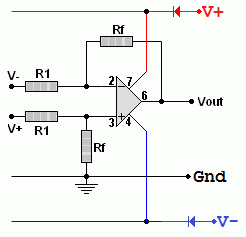Calculate Vout Vout = ( V+ - V- ) x ( Rf / R1 ) Input Resistance 2 x R1 AS A2 Voltage Follower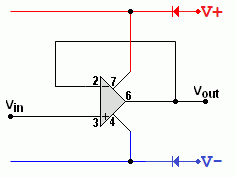Voltage Gain The voltage gain is ONE. Power and Current Gain This can be very large. Input resistance Infinity Output resistance Small so usefully large currents can be provided. A2 Schmitt Trigger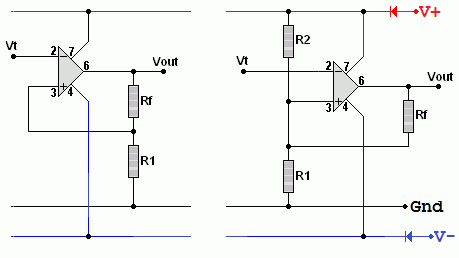On the left, a simple version with positive feedback giving different on and off reference voltages. On the right is a comparator with one extra resistor giving positive feedback to give different on and off reference voltages.